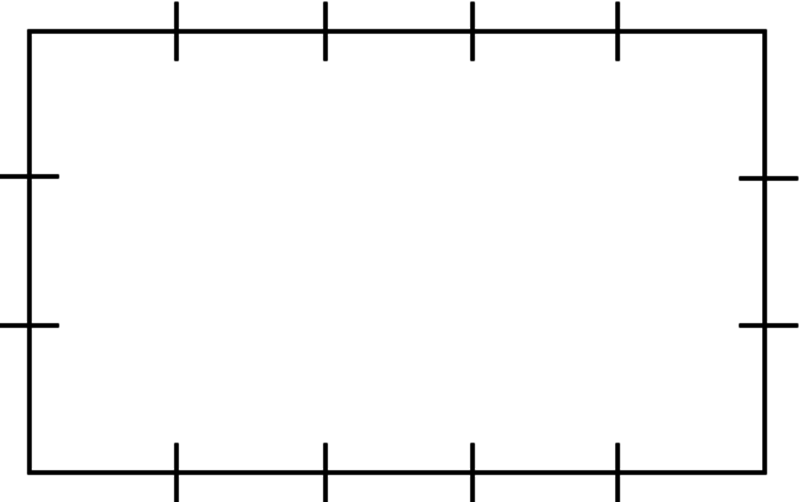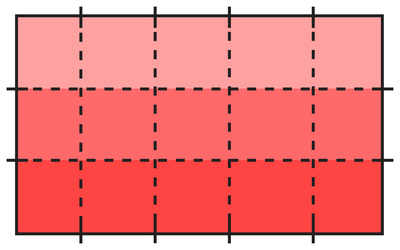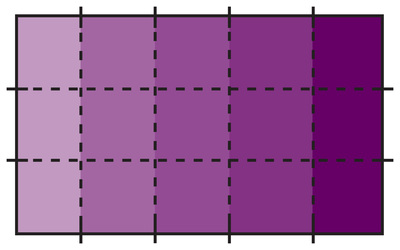Partitioning a Rectangle into Unit Squares

Alignments to Content Standards: 2.G.A.2 2.OA.C.4

Materials

• Copies of a rectangle with edges marked (one for each student/ group, see attached blackline master)

• A straight edge tool

Actions

The teacher should guide students through these actions, as the text in this task is too complex for some second graders.

1. Draw a grid on the rectangle by connecting each mark to the one directly across from it on the opposite edge.2. The grid separates the rectangle into many little squares. How many squares are there?

3. There are five little squares in each row. Count by fives to find how many squares there are in the entire rectangle.

4. What other methods can you think of to quickly count how many squares there are in the entire rectangle?

5. Write a number in each little square to count them and show that your answers are correct.

6. One number sentence which shows the total number of squares is 3 + 3 + 3 + 3 + 3 = 15.  Write another number sentence which shows the total number of squares.

IM Commentary

The purpose of this task is to show the student that a rectangle can be partitioned into unit squares, and that there are a number of reasonable ways to count the resulting squares.

The third part encourages the student to count by fives, as called for by 2.NBT.2, and makes the connection between the equal-sized groups and area of a rectangle representations for multiplication, which will be developed in third grade. Counting by numbers other than five is not called for by the standards, but would be a nice additional outcome.

Many extensions are possible:

• Inviting students to make their own rectangle with a grid of a size of their choosing.

• To learn about world cultures as well as mathematics, one can take the opportunity to explore game boards with this kind of shape. In particular, Snakes and Ladders is a game which has been played around the world. (Commercially, Chutes and Ladders in the U.S. is based on it). See the Wikipedia page on Snakes and Ladders for more information.

Students could make their own game with different-sized rectangular boards and different ways to navigate them, reinforcing the fact that there are different ways to count and all ways give the same answer (connecting 2.G.A.2 with K.CC.4.B). Playing such games can develop skills in estimation, the relationship between multiplication and division, and probability, setting the ground for a wide range of later work.

• Investigating which numbers can be represented as the total number of squares in some grid. Even at this grade level, some students may take an interest in numbers which can only be made by a grid with side length one (that is, prime numbers).

Students need to experience partitioning rectangles themselves to understand area. If students have not yet done this, then they should do the partitioning themselves. However, if the students are already proficient with partitioning and understand that the squares taken together constitute the larger rectangle, then they can start with a pre-partitioned rectangle.

Solution

1.2. For this particular example, there are fifteen little squares.

3. There are five little squares in each row. To find the total amount, the student can count by fives: five, ten, fifteen. Since the student has already counted that there are a total of fifteen squares, they can feel confident that counting by fives is giving them the correct number of squares. Compare with standard 2.OA.C.44. The student might also notice that there are three little squares in each column. To find the total amount, the student can count by threes: three, six, nine, twelve, fifteen. Counting by numbers other than five, while not strictly necessary in a task at this grade level, nonetheless provides a foundation for working on multiplication in third grade.5. Students can label the squares in different ways. In particular, it might be helpful for the student to make a few copies of their grid and try a few different labeling schemes.

To reinforce counting by the number of squares in each column, the teacher might encourage the student to label the top row with the numbers 1 through 5, the second row with the numbers 6 through 10, and the third row with the numbers 11 through 15.

To reinforce counting by the number of squares in each column, the teacher might encourage the student to label the leftmost column with the numbers 1 through 3, the second column with the numbers 4 through 6, and so forth.

6. Another number sentence which can be used is 5 + 5 + 5 = 15.  While others are possible, including for example 9 + 6 = 15 (breaking it up into a familiar square an six more blocks) and 1 + 1 + ... + 1 = 15, number sentences which describe breaking up by rows or columns are the desired and most likely responses.